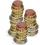# Multiplication principle + divisibility - math problems

#### Number of problems found: 10

• CombinationsHow many different combinations of two-digit number divisible by 4 arises from the digits 3, 5 and 7?
• How many 4How many 4 digit numbers that are divisible by 10 can be formed from the numbers 3, 5, 7, 8, 9, 0 such that no number repeats?
• No. of divisorsHow many different divisors have number ??
• DigitsHow many odd four-digit numbers can we create from digits: 0, 3,5,6,7?
• Three-digit integersHow many three-digit natural numbers exist that do not contain zero and are divisible by five?
• Twenty-fiveHow many three-digit natural numbers are divisible by 25?
• StacksAnnie has a total of \$ 702. The money must be divided into stacks so that each buyer has the same amounth of dollars. How many options she have?
• Three digits numberFrom the numbers 1, 2, 3, 4, 5 create three-digit numbers that digits not repeat and number is divisible by 2. How many numbers are there?
• RectanglesHow many rectangles with area 8713 cm2 whose sides are natural numbers are?
• Inverted nineIn the hotel,, Inverted nine" each hotel room number is divisible by 6. How many rooms we can count with three-digit number registered by digits 1,8,7,4,9?

We apologize, but in this category are not a lot of examples.
Do you have an interesting mathematical word problem that you can't solve it? Submit a math problem, and we can try to solve it.

We will send a solution to your e-mail address. Solved examples are also published here. Please enter the e-mail correctly and check whether you don't have a full mailbox.

Please do not submit problems from current active competitions such as Mathematical Olympiad, correspondence seminars etc...

Multiplication principle - math word problems. Divisibility - math word problems.